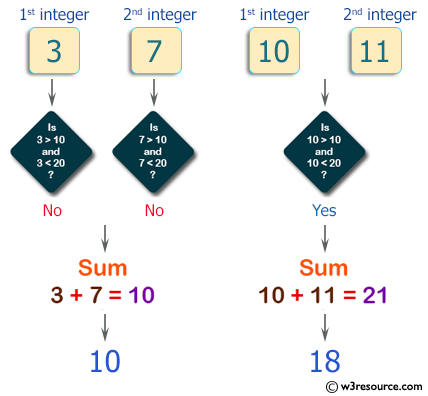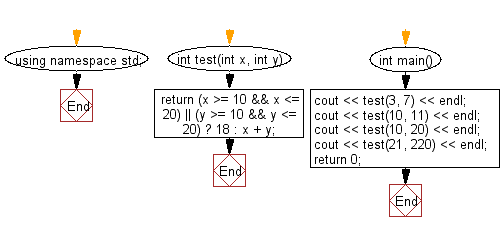﻿ C++ : Sum 2 integers, if one is in range 10..20, return 18# C++ Exercises: Compute the sum of the two given integers, if one of the given integer value is in the range 10..20 inclusive return 18

## C++ Basic Algorithm: Exercise-42 with Solution

Write a C++ program to compute the sum of the two given integers. If one of the given integer value is in the range 10..20 inclusive return 18.

Sample Solution:

C++ Code :

``````#include <iostream>

using namespace std;

int test(int x, int y)
{
return (x >= 10 && x <= 20) || (y >= 10 && y <= 20) ? 18 : x + y;
}

int main()
{
cout << test(3, 7) << endl;
cout << test(10, 11) << endl;
cout << test(10, 20) << endl;
cout << test(21, 220) << endl;
return 0;
}
``````

Sample Output:

```10
18
18
241
```

Pictorial Presentation:Flowchart:C++ Code Editor: